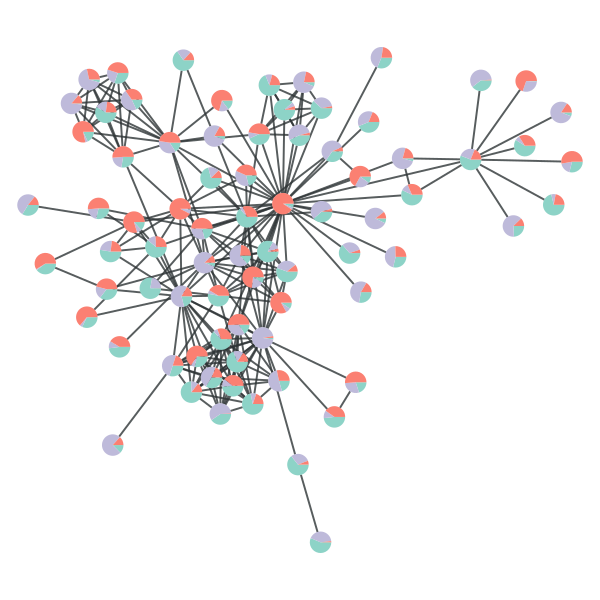# How do I make a different pie chart for each vertex?

Hi,
I have troubles understanding the "vertex_pie_fractions" parameter in
graph_draw.
I would like to draw different pie charts for each of the n vertices of a
graph.
In my case, each pie must be divided in two fractions.
I try to assign to the "vertex_pie_fractions" parameter a nx2 list, or an
equivalent numpy array with no success.

I wonder if it is possible to have a different pie chart for each vertex, or
if I'm wrong in coding.

Luigi

P.S. Yes, I do first the assignment "vertex_shape="pie"".

You have to use a property map. For example:

g = collection.data["lesmis"]
pie_fracs = g.new_vertex_property("vector<double>")

for v in g.vertices():
pie_fracs[v] = numpy.random.dirichlet([1, 1, 1])

graph_draw(g, pos=g.vp["pos"], vertex_shape="pie", vertex_pie_fractions=pie_fracs, output="pie.png")

You can see the result attached.

Best,
TiagoThanks Tiago, you are really helpful.

Best regards,
Luigi